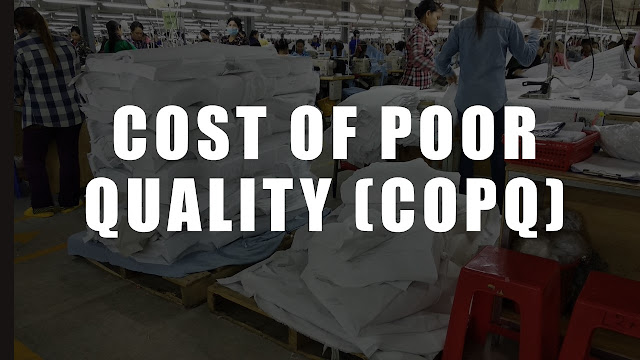# How to Calculate Cost of Poor Quality (COPQ) in Readymade Garment Manufacturing

In an earlier post, I have discussed the cost of poor quality in the apparel industry. If you missed that post read it here.

I have visited many readymade garment manufacturers and worked on quality improvement projects with numerous companies. I observed none of those garment factories measured the cost of poor quality for the internal failures, appraisal cost and preventive cost of quality.Now you learned that you can measure how much money you are wasting due to managing quality poorly in your factory. By measuring COPQ you can truly know how much money you are wasting. This is an opportunity for improvement.

In the previous article, we have discussed four kinds of cost of poor quality - internal failure, external failure, appraisal cost and preventive cost. In this article, I will show you how you can calculate internal failure cost, appraisal cost and preventive cost for making quality garments.

Internal failure costs are incurred due to rework, repair of defective garments and rejection of garments and raw material.

Also See: Importance of Quality Control in Garment Manufacturing

### Steps to calculate COPQ

Step-1: You first need to measure the amount of rework, and rejection level. Amount of rework can be measured through DHU and percentage defectives. From the DHU value, calculate total number of defects need to be repaired daily (A).

Step-2: Study how much time you need to remove stitches from a defective garment due to stitching defects (T1)

Step-3: Study how much time (average time) operators take to re-stitch the garment (T2). Defect wise repair time would vary.

Step-4: Measure cost of manpower for mending, spotting, and repair work. It would equal number manpower involved in rework multiply by their daily wages.

To find rework labour requirement use this formula
Number of labour needed for repair work (L) = (A * (T1+T2))/480
Here one labour is equivalent to 480 minutes.
Then calculate it into extra wages for excess labour hours used in repair works from daily average salary.
Labour cost in repair work = L * Wages per day per labour

Step-5: Excess fabric consumed - If you need to change garment parts, how much extra fabric is issued for re-cutting. Calculate cost of excess fabric (FC).

Step-6: Calculate cost of re-cutting (manpower cost) in case re-cutting/additional cutting is done (CC).

Step-7: Calculate Rejection cost: Calculate how many garments are rejected (percentage of total pieces of an order). Calculate total loss of potential FOB earning.

Step-8: Find internal failure cost from salary of alteration tailors + Cost of rejected garments + cost of excess material usage (FC) + re-cutting cost (CC)

Step-9: Calculate total COPQ per month = (rework cost + rejection cost+ excess manpower cost+ cost of quality team)

Step-10: Value of total shipments per month

Step-11: Calculate COPQ percentage of total shipment value

### An example of the Cost of Poor Quality calculation:

This example is shown with hypothetical data for calculating COPQ.

Internal failure cost: A factory stitching quality is measured as DHU 10 and in finishing DHU 15 and produced 1000 pieces per day.

Therefore total number of defects in 1000 piece is 250 (stitching + finishing).

To ease the calculation let consider each defect take same amount of time to repair. Assume that it takes 5 minutes per defects to repair. Therefore you will be needed 1250 man minutes to repair all defects. This is equal to 2.6 man-days. So monthly man-days required for repair work (2.6 * 25)

Average wages of workers is Rs. 7,000/-

So monthly internal failure cost due to repair work = 2.6 *7000 = Rs. 18,200/- Monthly

Rejection cost: After finishing you found 1% garment rejection of the order quantity. It would be equal to 10 garments per day. Calculate loss of money due to pieces rejected based on FOB per pieces (you may debate on this why FOB?) Alternatively, you can only consider material cost and labour cost incurred to process those 10 pieces till it get damaged. I am considering FOB to calculate potential revenue loss. FOB is \$5, therefore loss due to garment rejection = 10*\$5 = \$50 = Rs. 3250/- Daily (\$1=INR 65) and Rs 81,250 Monthly (25 days a month)

Appraisal cost: Cost of personnel involved in checking, auditing

Checking cost: Assume that factory have following team for the quality inspection, checking and managing quality department.

• Fabric checker: 2 no,
• Cutting room checker: 1 no.
• Stitching section checker including inline, end-of-line: 4 no.
• Finishing checker: 4 no. and

Assume salary of the quality head is Rs 30,000 per month and checker’s salary is Rs. 7,000 per day. Calculate total appraisal cost from salary of the quality team. It is 'Rs. (30,000 + 11*7,000) = Rs. 107,000 Monthly

Considering only internal failure cost and appraisal cost, cost of poor quality of the factory is equal to (Rs. 18,200 + Rs. 81,250 + Rs. 107,000) = Rs. 206,450 Monthly

Total FOB value of 25,000 pieces = 25,000 *\$5*65 = Rs. 81, 25,000

Cost of poor quality in Percentage = (Rs. 206,450/ Rs. 81, 25, 000)*100 = 2.54%

P.S.: To be noted that this method is just for your reference to calculate the cost of poor quality. You may not get the accurate value of COPQ. You can extend data collection and calculate the accurate cost of poor quality by detailed analysis.

P.S.S: Cost of poor quality is not only the repair work but there is external failure cost too. You can include external failure to find the total cost of poor quality.

Read more articles on Quality Control

1.